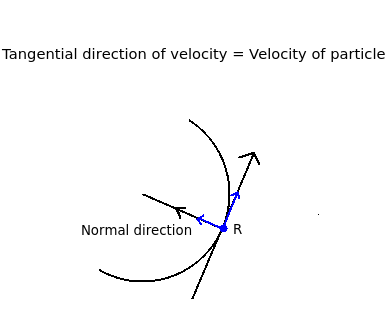# Engineering Mechanics - Mechanical Engineering (MCQ) questions and answers for Q. 11356

Q.  If a particle moves along a curved path, then what will be the normal component of velocity acting on the particle, if magnitude of tangential component of velocity is 30 m/s at an angle of 50o with the vertical?
- Published on 21 Sep 15

a. 57.8 m/s
b. 68.9 m/s
c. 40.2 m/s
d. None of the above

#### Discussion

• Sravanthi   -Posted on 15 Dec 15
- Curvilinear motion: This type of motion occurs when an object moves in a curved path.

- The velocity of a particle moving in a curved path is always tangential to the path moved. Hence, the tangential component of velocity is equal to velocity itself as the particle moves tangentially and the normal component is zero.

- The normal component is perpendicular to the velocity vector and passes through the centre of curvature. This type of co-ordinate system, in which motion of particle is described in two directions is known as normal and tangential co-ordinate system.

- R is the particle moving in a curved path shown in the diagram.

- Hence, Vnormal component = 0 and Vtangential component = velocity of the particle## ➨ Post your comment / Share knowledgeEnter the code shown above:

(Note: If you cannot read the numbers in the above image, reload the page to generate a new one.)